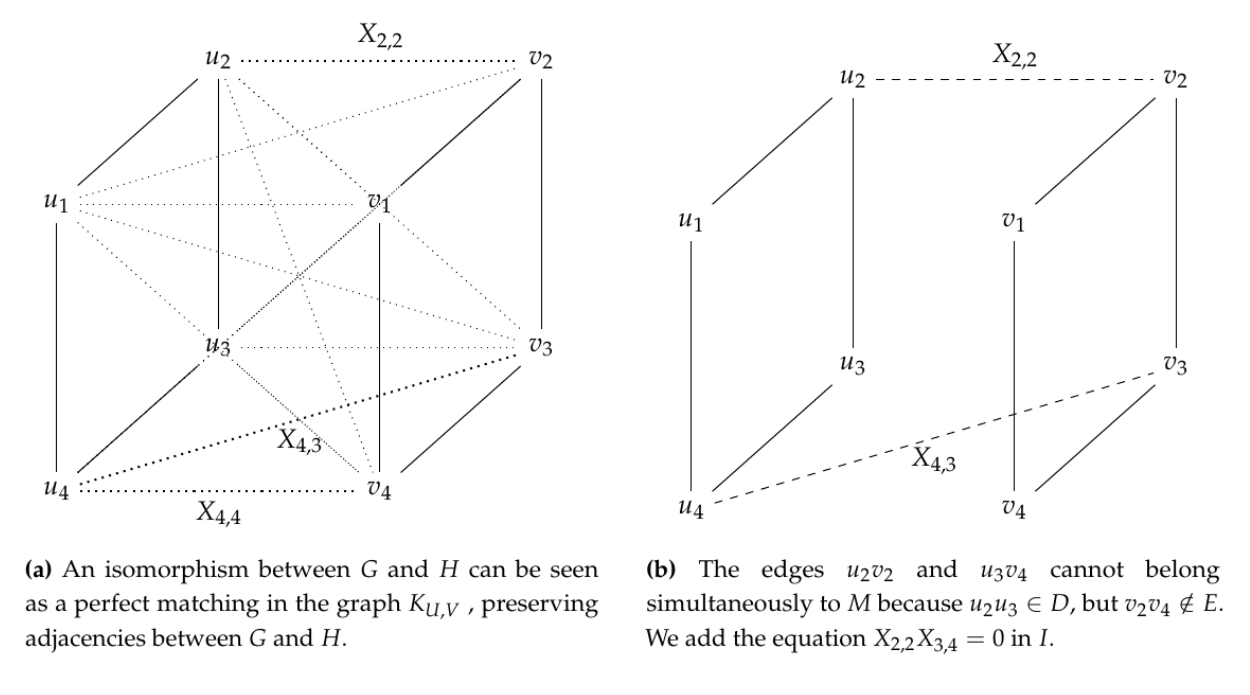Preprint Article Version 1 Preserved in Portico This version is not peer-reviewed

# A Zero-Knowledge Proof Based on a Multivariate Polynomial Reduction of the Graph Isomorphism Problem

Version 1 : Received: 7 May 2018 / Approved: 8 May 2018 / Online: 8 May 2018 (09:35:15 CEST)

How to cite: González Fernández, E.; Morales-Luna, G.; Sagols Troncoso, F. A Zero-Knowledge Proof Based on a Multivariate Polynomial Reduction of the Graph Isomorphism Problem. Preprints 2018, 2018050126 (doi: 10.20944/preprints201805.0126.v1). González Fernández, E.; Morales-Luna, G.; Sagols Troncoso, F. A Zero-Knowledge Proof Based on a Multivariate Polynomial Reduction of the Graph Isomorphism Problem. Preprints 2018, 2018050126 (doi: 10.20944/preprints201805.0126.v1).

## Abstract

Zero-Knowledge Proofs ZKP provide a reliable option to verify that a claim is true without giving detailed information other than the answer. A classical example is provided by the ZKP based in the Graph Isomorphism problem (GI), where a prover must convince the verifier that he knows an isomorphism between two isomorphic graphs without publishing the bijection. We design a novel ZKP exploiting the NP-hard problem of finding the algebraic ideal of a multivariate polynomial set, and consequently resistant to quantum computer attacks. Since this polynomial set is obtained considering instances of GI, we guarantee that the protocol is at least as secure as the GI based protocol.## Keywords

graph isomorphism problem; multivariate polynomial system; zero-knowledge proof

## Subject

MATHEMATICS & COMPUTER SCIENCE, Computational Mathematics

Views 0